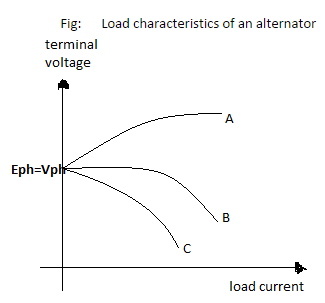# Voltage Regulation of an Alternator

## Voltage Regulation of an Alternator

Q1. The open circuit voltage and short circuit current of a 3 phase, star connected alternator are 1050 V and 250 A respectively. Its field current is 12 A. Then the synchronous impedance of alternator will be

a. 1.34 ohm
b. 1.69 ohm
c. 2.42 ohm
d. 2.85 ohm

Q2. A 6 kVA, 220 v 3 phase, star connected synchronous generator has a winding resistance of 0.15 ohm per phase and synchronous reactance of 6.1 ohm per phase. The voltage regulation of alternator at a load power factor of 0.8 lagging will be

a. 58.10 %
b. 68.43 %
c. 69.02 %
d. 72.81 %

Q3. If two mechanically coupled alternators deliver power at 50 Hz and 60 Hz respectively, then the highest speed of alternators will be

a. 1200 rpm
b. 1500 rpm
c. 600 rpm
d. 300 rpm

Q4. If the armature resistance and synchronous reactance of a 2 MVA, 11 kV, 3 phase star connected alternator are 2 ohm and 20 ohm per phase respectively. The value of full load generated voltage per phase at unity power factor will be

a. 6888.45 V
b. 6899.65 V
c. 6999.45 V
d. 7111.45 V

Q5. As the value of load power factor of an alternator changes, the value of armature reaction reactance

a. Remains constant
b. Changes

Q6. The given figure shows the load characteristics of an alternator. Which among the following is correct?a. A – Leading p.f., B – unity p.f., C – lagging p.f.
b. A – Lagging p.f., B – unity p.f., C – leading p.f.
c. A – Leading p.f., B – lagging p.f., C – unity p.f.
d. A – Unity p.f., B – leading p.f., C – lagging p.f.

ANSWER: a. A – Leading p.f., B – unity p.f., C – lagging p.f.

Q7. In an alternator, the voltage regulation will be positive when the power factor of the load is

b. Unity
c. Lagging
d. Both ( b ) & ( c )

ANSWER: d. Both ( b ) & ( c )

Q8. On unity power factor, the terminal voltage of an alternator is

a. Always less than induced emf
b. Always greater than induced emf
c. Same as induced emf
d. None of these

ANSWER: a. Always less than induced emf

Q9. The percentage voltage regulation of an alternator is given by

A. {(Eph - Vph/ Eph) *100}
B. {(Vph - Eph / Eph)*100}
C. {(Eph - Vph / V ph)*100}
D. {(Vph - Eph / V ph)*100}

ANSWER: C. {(Eph-Vph / V ph)*100}